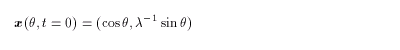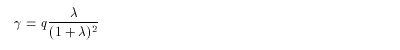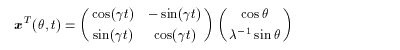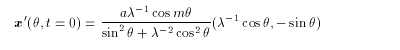2. Theory
2.1 Contour Dynamics
2.2 Equations of Cotour Dynamics
2.3 Theory of an Elliptical Vortex
2.4 Finite-Time Lyapunov Analysis on Fluid Deformation

### 2.3 Theory of an Elliptical Vortex

In two-dimentional nondivergent perfect fluid, an ellipse is assumed as(2.12)
where λ is the ratio of the major axis to the minor axis, and the initial vorticity is given to be a constant q inside the ellipse and 0 outside.

Such a vortex rotates with a constant angular velocity g, depending on l and q ( Lamb,1932 ):(2.13)(2.14)

Love(1893) examined the stability of the vortex when the following small perturbation is added:(2.15)

• When m = 1 , the perturbation is always neutral.
• When m = 2 , the perturbation is always neutral and does not change in time.
• When m = 3 , the perturbation grows in time and the vortex is unstable if l > 3 .

<<index <previous next>